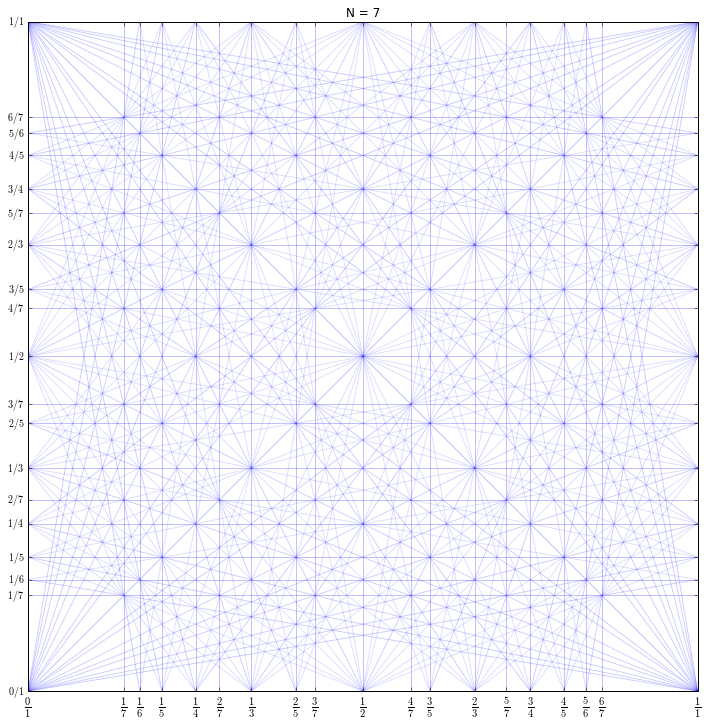# jorgeh@ccrma

## Resonant Sequences

Sat 25 April 2015

# Resonant Sequences¶

In :
!date

Thu Apr  9 15:28:26 PDT 2015

In :
from __future__ import division
import math

def fareySequence(N, k=1):
"""
Generate Farey sequence of order N, less than 1/k
"""
# assert type(N) == int, "Order (N) must be an integer"
a, b = 0, 1
c, d = 1, N
seq = [(a,b)]
while c/d <= 1/k:
seq.append((c,d))
tmp = int(math.floor((N+b)/d))
a, b, c, d = c, d, tmp*c-a, tmp*d-b
return seq

def resonanceSequence(N, k):
"""
Compute resonance sequence

Arguments:
- N (int): Order
- k (int): denominator of the farey frequency resonances are attached to
"""
a, b = 0, 1
c, d = k, N-k
seq = [(a,b)]
while d >= 0:
seq.append((c,d))
tmp = int(math.floor((N+b+a)/(d+c)))
a, b, c, d = c, d, tmp*c-a, tmp*d-b
return seq

def plotResonanceDiagram(N, figsize=(10,10)):
import matplotlib.pyplot as plt

ALPHA = 0.5/N

plt.figure(figsize=figsize)
ticks = set([])
for h, k in fareySequence(N, 1):
for a, b in resonanceSequence(N, k):
if b == 0:
x = np.array([h/k, h/k])
y = np.array([0, 1])
elif a== 0:
x = np.array([0, 1])
y = np.array([h/k, h/k])
else:
m = a/b
cp, cm = m*h/k, -m*h/k
x = np.array([0, h/k, 1])
y = np.array([cp, 0, cm+m])
plt.plot(  x,   y, 'b', alpha=ALPHA) # seqs. attached to horizontal axis
plt.plot(  y,   x, 'b', alpha=ALPHA) # seqs. attached to vertical axis
# also draw symetrical lines, to be fair (otherwise lines in the
# lower left traingle will be duplicated, but no the others)
plt.plot(  x, 1-y, 'b', alpha=ALPHA)
plt.plot(1-y,   x, 'b', alpha=ALPHA)
plt.xlim(0, 1)
plt.ylim(0, 1)
plt.xticks([h/k for h,k in ticks], [r"$\frac{{{:d}}}{{{:d}}}$".format(h,k) for h,k in ticks], fontsize=15)
plt.yticks([h/k for h,k in ticks], [r"${:d}/{:d}$".format(h,k) for h,k in ticks])
plt.title("N = {:d}".format(N))


## Generating resonance sequences is fast¶

Try it!

Note: in the original paper there was a minor mistake. Eq. (8) read

$$\Bigg( \Big\lfloor \frac{N+b+a}{d} \Big\rfloor c - a , \Big\lfloor \frac{N+b+a}{d} \Big\rfloor d - b \Bigg)$$

but it should read

$$\Bigg( \Big\lfloor \frac{N+b+a}{d+c} \Big\rfloor c - a , \Big\lfloor \frac{N+b+a}{d+c} \Big\rfloor d - b \Bigg)$$

I've contacted Rogelio Tomás (the author) and he agreed with the correction (an erratum will be sent to the publication).

In :
N = 5
for k in set([k for _,k in fareySequence(N, 1)]):
print "N={}, k={}:".format(N, k)
print "\t", resonanceSequence(N, k)

N=5, k=1:
[(0, 1), (1, 4), (1, 3), (1, 2), (2, 3), (1, 1), (3, 2), (2, 1), (3, 1), (4, 1), (1, 0)]
N=5, k=2:
[(0, 1), (2, 3), (2, 2), (2, 1), (4, 1), (2, 0)]
N=5, k=3:
[(0, 1), (3, 2), (3, 1), (3, 0)]
N=5, k=4:
[(0, 1), (4, 1), (4, 0)]
N=5, k=5:
[(0, 1), (5, 0)]


## ..., but plotting can be slow for large N (N > 10)¶

Try it, but be patient ... (lots of lines to plot)

In :
%matplotlib inline
# from matplotlib2tikz import save as save_tikz
import numpy as np
import matplotlib.pyplot as plt
plotResonanceDiagram(7, figsize=(12,12))

# save_tikz('resonanceDiagram_N7.tikz')